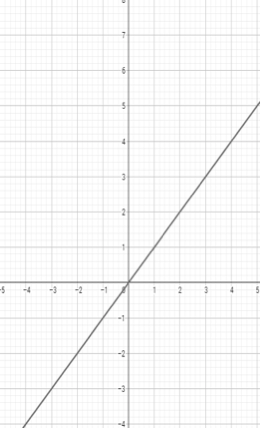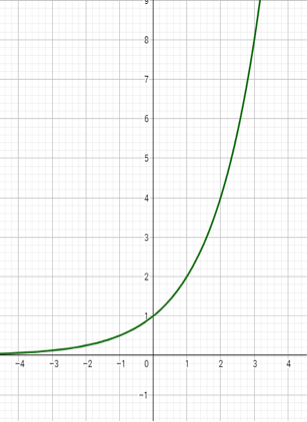# FNGR 1 | Lesson 4 | Explore (Linear, Exponential and Quadratic Functions)# Linear, Exponential and Quadratic Functions

When a function is shifted horizontally or vertically it is said to be transformed. As we’ve seen, functions come in many types and names.  In the table below are the parent functions we’ve been studying in these modules. Graphs are created using Desmos.com.

$$y=x$$ $$y=x^2$$ $$y=2^x$$